Published

OGC Engineering Report

Testbed-18: 3D+ Data Space Object Engineering Report
Martin Desruisseaux Editor Logan Stark Editor
OGC Engineering Report

Published

 Document number: 23-011r1 Document type: OGC Engineering Report Document subtype: Document stage: Published Document language: English

Use of this document is subject to the license agreement at https://www.ogc.org/license

# I.  Executive Summary

This OGC Testbed 18 3D+ Data Space Object Engineering Report (ER) begins with the application of ISO 19111: Geographic Information – Spatial referencing by coordinates (ISO 19111:2019) to the reference frame of objects in space such as celestial bodies or spacecraft in orbit. The Data Space Object ER sits between the 3D+ Standards Framework Engineering Report (OGC 22-036), which introduces the theoretical grounds, and the Reference Frame Transformation Engineering Report (OGC 22-038), which applies ISO 19111 to coordinate operations between above-cited frames. This ER explores the flexibility of the ISO 19111 model and concepts for adapting the coordinate system to the object geometry (cylindrical, triaxial ellipsoid, etc.).

Coordinate Reference Systems (CRSs) are sometimes inseparable from the coordinate operation that relates them to another system. This is the case of the ISO 19111 concept DerivedCRS class where by definition a CRS is specified in terms of its relationship with another CRS. This situation is also the case in the OGC GeoPose 1.0 Data Exchange standard (OGC 21-056r10) which describes a chain of inner frames related to outer frames by coordinate operations. Due to this interdependency, both derived CRS and GeoPose are discussed in OGC 22-038.

According to this ER, the ISO 19111 Standard has the richest set of capabilities for expressing complex chain of coordinate operations while preserving information about changes of datum. The latter are constraints of the physical world. Most concepts developed in GeoPose Standard and in NASA SPICE software can be mapped to ISO 19111 concepts. GeoPose provides a more compact encoding for the use cases of interest to GeoPose.

# II.  Keywords

The following are keywords to be used by search engines and document catalogues.

ogcdoc, TB-18, CRS, 3D+, space-time

# III.  Security considerations

No security considerations have been made for this document.

# IV.  Submitting Organizations

The following organizations submitted this Document to the Open Geospatial Consortium (OGC):

• Blue Monocle, Inc.
• Geomatys

# V.  Contributors

Editors and contributors to this Document include the following:

• Martin Desruisseaux (Geomatys) — editor

• Charles Heazel (Heazeltech) — contributor

• Logan Stark (Blue Monocle, Inc.) — editor

# VI.  Abstract

With the growing commercialization of space there is a need to look beyond the earth and explore the integration of sensors or assets in celestial orbits or in free flight in our solar system. Their exact tracking and localization are becoming increasingly important as space emerges as the newest area in need for standard-based mechanisms for streaming and for data integration from various sensors.

This Open Geospatial Consortium (OGC) Testbed 18 3D+ Data Space Object Engineering Report (ER) describes existing standards in terms of their ability to represent a suite of multidimensional Coordinate Reference Systems (CRS) and associated geometries as well as identifies shortfalls in these standards.

Testbed-18: 3D+ Data Space Object Engineering Report

# 1.  Scope

This OGC Testbed 18 Engineering Report provides information about the representation of coordinate reference systems (CRSs) using existing standards. The ER describes how the frame definition can be adapted to different kinds of object geometry such as cylinders. The ER does not cover the transformations between two or more reference frames. This topic is covered by the Reference Frame Transformation Engineering Report (OGC 22-038). The theoretical grounds for reference frames are covered in the OGC 3D+ Standards Framework Engineering Report (OGC 22-036).

# 2.  Normative references

The following documents are referred to in the text in such a way that some or all of their content constitutes requirements of this document. For dated references, only the edition cited applies. For undated references, the latest edition of the referenced document (including any amendments) applies.

ISO: ISO 19107:2003, Geographic information — Spatial schema. International Organization for Standardization, Geneva (2003). https://www.iso.org/standard/26012.html.

ISO: ISO 19109:2015, Geographic information — Rules for application schema. International Organization for Standardization, Geneva (2015). https://www.iso.org/standard/59193.html.

ISO: ISO 19111:2019, Geographic information — Referencing by coordinates. International Organization for Standardization, Geneva (2019). https://www.iso.org/standard/74039.html.

ISO: ISO 19136:2007, Geographic information — Geography Markup Language (GML). International Organization for Standardization, Geneva (2007). https://www.iso.org/standard/32554.html.

ISO: ISO 19141:2008, Geographic information — Schema for moving features. International Organization for Standardization, Geneva (2008). https://www.iso.org/standard/41445.html.

ISO: ISO 19162:2019, Geographic information — Well-known text representation of coordinate reference systems. International Organization for Standardization, Geneva (2019). https://www.iso.org/standard/76496.html.

Carl Stephen Smyth: OGC 21-056r10, OGC GeoPose 1.0 Data Exchange Draft Standard. Open Geospatial Consortium (2022). https://docs.ogc.org/dis/21-056r10/21-056r10.html.

# 3.  Terms, definitions and abbreviated terms

This document uses the terms defined in OGC Policy Directive 49, which is based on the ISO/IEC Directives, Part 2, Rules for the structure and drafting of International Standards. In particular, the word “shall” (not “must”) is the verb form used to indicate a requirement to be strictly followed to conform to this document and OGC documents do not use the equivalent phrases in the ISO/IEC Directives, Part 2.

This document also uses terms defined in the OGC Standard for Modular specifications (OGC 08-131r3), also known as the ‘ModSpec’. The definitions of terms such as standard, specification, requirement, and conformance test are provided in the ModSpec.

For the purposes of this document, the following additional terms and definitions apply.

## 3.1. coordinate

one of a sequence of numbers designating the position of a point

Note 1 to entry: In a spatial coordinate reference system, the coordinate numbers are qualified by units.

[SOURCE: ISO 19111:2019]

## 3.2. coordinate reference system

coordinate system that is related to an object by a datum

Note 1 to entry: Geodetic and vertical datums are referred to as reference frames.

Note 2 to entry: For geodetic and vertical reference frames, the object will be the Earth. In planetary applications, geodetic and vertical reference frames may be applied to other celestial bodies.

[SOURCE: ISO 19111:2019]

## 3.3. coordinate tuple

tuple composed of coordinates

Note 1 to entry: The number of coordinates in the coordinate tuple equals the dimension of the coordinate system. The order of coordinates in the coordinate tuple is identical to the order of the axes of the coordinate system.

[SOURCE: ISO 19111:2019]

## 3.4. earth-centered inertial

a reference frames having its origin at the center of mass of Earth and which is fixed with respect to the stars

Note 1 to entry: For objects in space, the equations of motion that describe orbital motion are simpler in a non-rotating frame such as ECI.

## 3.5. earth-fixed

a reference frame which remains fixed with respect to Earth’s surface in its rotation, and then rotates with respect to stars

Note 1 to entry: Geodetic CRS defined by ISO 19111 are Earth-Fixed.

## 3.6. engineering coordinate reference system

coordinate reference system based on an engineering datum

Note 1 to entry: For example, a coordinate reference system local to a moving object such as a ship or an orbiting spacecraft.

[SOURCE: ISO 19111:2019]

## 3.7. engineering datum

datum describing the relationship of a coordinate system to a local reference

Note 1 to entry: Engineering datum excludes both geodetic and vertical reference frames.

[SOURCE: ISO 19111:2019]

## 3.8. reference frame

parameter or set of parameters that realize the position of the origin, the scale, and the orientation of a coordinate system

[SOURCE: ISO 19111:2019]

## 3.9. Minkowski spacetime

a combination of three-dimensional Euclidean space and time into a four-dimensional manifold where the spacetime interval between any two events is independent of the inertial frame of reference in which they are recorded

## 3.10.  Abbreviated terms

CRS

Coordinate Reference System

CS

Coordinate System

CSV

Comma Separated Values

ECEF

Earth-Centered — Earth-Fixed

ECI

Earth-Centered Inertial

GML

Geographic Markup Language

ICRF

International Celestial Reference Frame

WKT

Well-Known Text

1D

One-dimensional

3D

Three-dimensional

4D

Four-dimensional

# 4.  Introduction

Currently, most OGC Standards focus on data that is observed on the surface or directly above planet Earth. Other standards, such as GeoSciML, look into the planet and provide a data model and transfer standard for geological data. There has been less focus on extra-terrestrial space and the exact location of remote sensors. The goal of this Engineering Report (ER) is to evaluate the current standards and standard-based mechanisms used to describe location, trajectory, and orientation of objects in orbit or free flight in our solar system. A separate OGC Testbed 18 ER explores standard-based mechanisms to transform a location within a reference frame to a location within another reference frame (OGC 22-038). Another point of interest is to stream and integrate data from various sensors mounted on devices that are described in different reference frames and located anywhere in the solar system (OGC 22-035).

Data structures for reference frames and coordinate systems are defined by the ISO 19111:2019 – Referencing by Coordinates Standard (ISO 19111:2019). This joint OGC/ISO Standard is also published as an OGC Abstract Specification, Topic 2: referencing by coordinates. In most sections of the Data Space Object ER, descriptions of concepts are accompanied by descriptions of their relationships with ISO 19111. ISO 19111 terms will be shown in bold characters when first defined.

# 5.  Spatial CRS

An ISO 19111 Coordinate Reference System (CRS) is a combination of a reference frame (Clause 5.1) with a coordinate system (Clause 5.2), possibly completed by conversion parameters (typically map projections). The reference frame identifies the location and motion of CRS origin, while the coordinate system provides information about axes such as their units of measurement.

The relationship between CRS types and Reference Frame types is close to (but not exactly) a one-to-one relationship. For example, a GeodeticCRS is always associated with a GeodeticReferenceFrame. However, the relationship between CRS instances and Reference Frame instances is not a one-to-one relationship. There are possibly hundreds of CRS instances associated with the same Reference Frame instance. For example, there are hundreds of ProjectedCRS instances associated with the same “WGS 84” reference frame instance in the EPSG geodetic dataset. Those CRS instances differ by the map projection parameters, axis directions, or units of measurement.

The relationship between CRS types and Coordinate System types is more flexible. For example, a GeodeticCRS can be associated with an EllipsoidalCS, SphericalCS, or CartesianCS. This flexibility is discussed in Clause 5.2.

Thousands of reference systems have been specified for use around the world or in specific regions and for various purposes. The multitude of CRSs has led to the creation of the EPSG registry (EPSG) which provides unique codes for objects such as CRSs, ellipsoids, and datums. The EPSG registry also provides information about how to transform coordinates from one CRS to another. Those transformations are discussed in the Reference Frame Transformation OGC 22-038 ER.

## 5.1.  Reference frames

A reference frame represents the position of the origin, the scale, and the orientation of a coordinate system. However that information is not encoded directly in the reference frame. ISO 19111 reference frames are identified only by their names, sometimes accompanied by information such as ellipsoid axis lengths or temporal epoch. The information contained in ISO 19111 reference frames is usually not sufficient for transforming coordinates between different frames without the help of an external database such as EPSG. This limitation is by design, as it would be very difficult to achieve reference frame descriptions comprehensive enough for inferring accurate transformations in all cases. In the past, attempts were made, notably with a TOWGS84 data structure associated with each frame description. That data structure was a WKT element in Well-Known Text (WKT) 1 or a CS_WGS84ConversionInfo class in OGC 01-009 with 7 parameters: the rotation terms, translation terms, and a scale factor to apply on geocentric coordinates. The use of WGS84 as a hub for inferring arbitrary transformations is called early-binding. That approach is in contrast with the requirement to use an external database. This approach is called late-binding because no parameters are available until both source and target reference frames are known. These two approaches are not equivalent. There are Earth-based examples where the early-binding approach has unavoidable errors of a few meters while the late-binding approach is accurate to a few centimeters (Annex A). Consequently, it is not sufficient to define reference frames as standalone objects. An organization must also define a registry equivalent to EPSG with reference frame definitions together with late-binding information.

### 5.1.1.  Reference frame types

Spatial reference frames can be classified broadly into the three following classes (OGC 22-036).

• Space-fixed. This class includes the Earth-Centered Inertial (ECI) frames.

• Planet-fixed. This class includes the Earth-Centered — Earth-Fixed (ECEF) frames.

• Local system. This class includes frames attached to spacecrafts.

An inertial frame is non-rotating with respect to the stars. Its velocity may be non-zero. For example the Earth is orbiting around the Sun, but the frame acceleration is considered negligible. Truly inertial frames are difficult to achieve in practice, so the “inertial” word in this ER should be understood as “quasi-inertial”.

For objects in space, the equations of motion that describe orbital motions are simpler in inertial (space-fixed) frames such as Earth-centered inertial (ECI). By contrast, to represent the positions and velocities of terrestrial objects, it is more convenient to use Earth-centered — Earth Fixed (ECEF) coordinates such as latitude, longitude, and altitude.

Each of above-cited classes can be further subdivided. For example, both spatial-fixed and planet-fixed frames have “conventional (inertial or terrestrial) reference system” and “true (inertial or terrestrial) reference system” sub-classes. Those sub-divisions reflect which effects are taken into account, for example precession or nutation.

This hierarchy of reference frames is detailed in the Testbed 18 OGC 22-036 ER. This hierarchy is not reflected in the ISO 19111:2019 standard, except for a separation between planet-fixed frames (geodetic reference frame) and local systems (engineering datum).

### 5.1.2.  Local systems

A local reference system can be used for a local area on a planet surface or for a moving object such as a spacecraft. The OGC 22-036 ER subdivides local systems into different classes reflecting different choices of north or zenith directions. In ISO 19111:2019 the distinction is rather based on whether the local system is defined by a conversion from another, usually geodetic, coordinate reference system (CRS). This is often the case for a platform fixed on Earth, in which case the local system is a derived CRS. Conversely if the position of the local system is not defined in relationship with another system, for example if the local system is attached to an object in free flight, this is an engineering CRS. Note that the use of an engineering CRS does not mean that there is no relationship with another reference system, it only means that such relationship is not part of the CRS definition. The relationship may be empirical and based on observations having uncertainties.

## 5.2.  Coordinate systems

The mathematics that can be applied for computing geometric properties in a given space depends on the type of coordinate system (CS). Most standards or toolkits examined by OGC 22-036, in particular NASA SPICE (SPICE) and the OGC GeoPose Standard, assume a Cartesian CS. ISO 19111 is more flexible as it allows choosing a coordinate system that is more convenient for the object geometry. For example, a rotating wheel space station (a.k.a. von Braun wheel) could use a cylindrical CS.

Some coordinate system types specified in ISO 19111 are listed below. This list is non-exhaustive, as a few additional coordinate system types exist for specific kinds of reference frame. For example, ISO 19111 also defines TemporalCS and OrdinalCS types, which are not considered in this section.

The Coordinate Reference System (CRS) attached to a space station should be an EngineeringCRS, but the coordinate system associated to that engineering CRS can be any one from the above list except EllipsoidalCS. Below is an example in Well-Known Text (WKT) format applied to a rotating wheel space station.

`ENGINEERINGCRS["Von Braun wheel",  ENGINEERINGDATUM["Wheel rotation axis"],  CS[cylindrical, 3],  AXIS["distance (r)", awayFrom, ORDER, LENGTHUNIT["metre", 1]],  AXIS["bearing (U)", clockwise, BEARING, ORDER, ANGLEUNIT["degree",0.0174532925199433]],  AXIS["height (h)", up, ORDER, LENGTHUNIT["metre", 1]]]`

Figure 1 — Engineering CRS for a rotating wheel space station (WKT)

### 5.2.1.  Ellipsoidal CS special case

Ellipsoidal coordinate systems are special in that applying mathematics with these systems requires knowledge of the semi-major and semi-minor axis lengths and that information is not part of the EllipsoidalCS class. Rather, that information is part of the GeodeticReferenceFrame class, through its ellipsoid association. Consequently, EllipsoidalCS can hardly be used as a standalone object. Its use requires the GeodeticCRS object which contains the EllipsoidalCS object. This particular relationship implies that attempts to use EllipsoidalCS with EngineeringCRS would provide incomplete information, because an EngineeringCRS can only be associated to an EngineeringDatum and the latter does not contain the ellipsoid association.

Whether the above restriction is a problem or not depends on whether there is a need to associate EllipsoidalCS with an EngineeringCRS. In other words, whether spacecrafts or other non-geodetic objects with ellipsoidal shapes are envisioned. If there is this need, a possible change to ISO 19111 could be to move the ellipsoid association from GeodeticReferenceFrame to EllipsoidalCS. However, the reason why the ellipsoid is currently associated with GeodeticReferenceFrame is because it was considered useful to keep information about the ellipsoid in, for example, a geocentric CRS using a spherical or Cartesian coordinate system. That information would be lost if the ellipsoid were associated with EllipsoidalCS.

### 5.2.2.  Two-dimensional spherical CS

A spherical coordinate system is usually three-dimensional. However, the planetary domain sometimes needs to express spherical coordinates on a planet surface using only geocentric latitudes and longitudes, without a radius. If the surface is a sphere, then classical two-dimensional geographic coordinates can be used. However, if the surface is an ellipsoid, then the geocentric, geodetic, and astronomical latitudes differ (see OGC 22-036 figure 23). If the desired latitude is really geocentric rather than geodetic, then the coordinate system needs to be a SphericalCS rather than an EllipsoidalCS. In the current version of the ISO 19111 Standard, spherical coordinate systems must be three-dimensional. Therefore, a corrigendum is proposed which would support the definition of two-dimensional spherical coordinate systems. In the latter case, by convention, the point would be located on the planet surface. That surface corresponds to different radius values depending on the latitude. Computing those values requires information about the ellipsoid axes, which is another argument for keeping the ellipsoid association in GeodeticReferenceFrame (Clause 5.2.1) despite EllipsoidalCS being an apparently more natural location.

Note that the third-dimension (radius or ellipsoidal height) is relative to the planet center in a SphericalCS, while the third dimension is relative to the planet surface in an EllipsoidalCS. Consequently converting a two-dimensional coordinate tuple to a three-dimensional tuple on the planet surface can be done easily with EllipsoidalCS. The third coordinate is simply zero, regardless of the ellipsoid. But in the SphericalCS case, that conversion cannot be done without knowledge about the Ellipsoid.

### 5.2.3.  Triaxial ellipsoid

ISO 19111 supports definition of triaxial ellipsoids with a “semi-median axis” attribute in addition to the “semi-major axis” and “semi-minor axis” (or “inverse flattening”) attributes. Triaxial ellipsoids can be associated to the GeodeticReferenceFrame in the same way as biaxial ellipsoids. However, this is a recent feature added in ISO 19111:2019 and not many libraries support triaxial ellipsoids yet.

### 5.2.4.  Axis angles in affine CS

AffineCS is a generalization of CartesianCS where axes are not necessarily perpendicular. Like EllipsoidalCS, application of mathematics in AffineCS space requires additional information — the angles between pair of axes. The current ISO 19111 Standard does not provide this information directly. Encoding that information in axis directions is possible, but that solution would not be interoperable. For example, before the introduction of the BEARING keyword in WKT, axes of polar projections in the EPSG database were used to express directions such as “South along 90 deg East” instead of “East” or “North”.

Whether angle information is useful enough to be worth an addition in ISO 19111 is not yet determined. If this information is considered useful, a possible data structure could be an axisAngle attribute in the AffineCS class. The data type would be Angle and the occurrence would be 1 for a two-dimensional affine CS, or 3 for a three-dimensional affine CS. In the latter case the three angle values could be as below, in order:

• angle from first axis to second axis;

• angle from second axis to third axis; and

• angle from third axis to the first axis.

Implementation experiments would be required for checking if above proposal can work.

### 5.2.5.  Cartesian CS right-handiness

While the CartesianCS class does not need to specify axis angles, it is still useful to know if the coordinate system is left-handed or right-handed. For example, the NASA SPICE software requires Cartesian coordinate systems to be right-handed. There is currently no easy way to get this information into the ISO 19111 model. Software could infer this information from axis directions but this can be difficult if the coordinate system uses uncommon axis directions such as “away from” or if directions such as “up” are non-obvious (as it may be in space). If the axisAngle attribute mentioned in Clause 5.2.4 is added, its values would be fixed to ±90° but the value signs may give indication about right-handiness. Alternatively, an easier extension could be to add to the CartesianCS class an isRightHanded attribute of type Boolean.

## 5.3.  New spatial CRS types

The ISO 19111 model supports two of the three broad classes mentioned in Clause 5.1.1. They are in the two last rows of the following table. The class which is not yet supported is the space-fixed system. Adding this support to ISO 19111 model may be relatively easy by adding the inertial classes shown in the first table row.

Table 1 — Types of reference frames

System classISO 19111 reference frame classISO 19111 CRS class
Space-fixedInertialReferenceFrame (proposal)InertialCRS (proposal)
Earth-fixedGeodeticReferenceFrameGeodeticCRS
Local systemEngineeringDatumEngineeringRS

The new inertial classes would be similar to their geodetic counterparts. This is like the GeodeticCRS class being associated with CartesianCS, SphericalCS, and EllipsoidalCS. As such, InertialCRS should allow associations to at least CartesianCS and SphericalCS. Whether InertialCRS should also allow association to EllipsoidalCS is yet to be determined.

Figure 18 in the OGC 22-036 ER shows sub-divisions of the above broad classes: conventional, mean, true, etc. There is currently no construct in the ISO 19111 model for this level of sub-division detail. While useful for the understanding of a chain of coordinate transformations, they may not be necessary for their execution by a software application. Nevertheless, if there is a need to represent this classification within the ISO 19111 model, it could be done, for example, with some naming convention applied on the value of the name attribute of Reference Frame classes. Alternatively, if the information is considered important enough, a code list could be added.

# 6.  Spatiotemporal CRS

This chapter describes the use of ISO 19111 for combining time and space into one data point. Two different approaches are proposed, depending on whether the Newtonian physics is sufficient or if Einstein’s relativity must be taken in account.

## 6.1.  Galilean transformations

Newtonian physics, the physics of everyday life, served humankind well for almost 200 years. Galilean transformations, also called Newtonian transformations, relate the space and time coordinates (x, y, z, t) of two systems moving at a constant velocity relative to each other. Consider a reference frame F which is moving at velocity vₓ in respect to an observer at F′. Assuming that the axes of both reference frames are parallel and that the velocity is along x and x′ axes, then transformations from reference frame F to reference frame F′ are as below:

• t′ = t

• x′ = xvₓt

• y′ = y

• z′ = z

The fact that temporal coordinates (t) are independent of spatial coordinates (x, y, z) makes it possible to handle spatial and temporal components as two independent coordinate reference systems. This is the idea underlying the use of CompoundCRS in ISO 19111 for adding a temporal component to a spatial CRS. This separation is adequate for describing phenomena at speeds much smaller than the speed of light. Galilean transformations express the ideas that space and time are absolute, with measurements that are independent of the relative motion of the observer. This also implies that all speeds are relative, including the speed of light, which would depend on the observer motion.

## 6.2.  Lorentz transformation

The 1887 ether-wind experiment of Michelson and Morley demonstrated that the speed of light is independent of the reference frame. Lorentz transformations are used for transforming coordinates between reference frames while preserving the light speed invariance. Consider a reference frame F which is moving in respect to an observer at F′. Transformation from reference frame F to reference frame F′ requires a transformation that accounts for the effect of velocity. Central to this transformation is the Lorentz factor (γ) defined as:

$\gamma \left(v\right)=1/\sqrt{1-{\left(v/c\right)}^{2}}$  (1)

In each reference frame, an observer can use a local Cartesian coordinate system to measure lengths and a clock to measure time intervals. An event is something that happens at a point in space at an instant of time, or more formally, a point in spacetime. The transformations connect the space and time coordinates of an event as measured by an observer in each frame. In the reference frame F, the coordinates are represented by (x, y, z, t). In the other reference frame F′ moving at a velocity vₓ relative to F, the coordinates are represented by (x′, y′, z′, t′). In both reference frames the coordinate axes are parallel, and they remain mutually perpendicular. The relative motion vₓ is along the x and x′ axes and this section assumes that there is no motion along other axes. At t = t′ = 0, the origins in both reference frames are the same (x, y, z) = (x′, y′, z′) = (0, 0, 0). At any other spatiotemporal coordinates, the transformations are as below:

• t′ = γ⋅(tvₓx/c²)

• x′ = γ⋅(xvₓt)

• y′ = y

• z′ = z

The above equations imply length contraction and time dilation. In most cases those effects are negligible because γ is almost 1 and v²/c² approaches 0. However, when v is a significant percentage of c, those equations should be applied.

The Lorentz contraction effect is the shortening of an object along the direction of its motion relative to an observer. Dimensions in other directions are not contracted. For applying above set of equations in practice, the F′ reference frame must be rotated and translated so as to be co-linear with F.

### 6.2.1.  Length property in Moving Feature instances

Features (ISO 19109:2015) can contain an arbitrary number of properties. Some properties may be measurements of the length of something. Because of length contraction effects, the definition of linear properties in Moving Features must clarify how lengths are measured. The following are two possibilities.

• Given that the motion of a moving feature is described relative to some external CRS, the values of a linear property could be lengths as measured by an observer in that external CRS.

• Alternatively, moving features could express lengths as proper lengths. A proper length is the length as observed by an observer moving with the moving feature. This is equivalent to defining an EngineeringCRS for each Moving Feature instances and declaring that length measurements are relative to that CRS.

The second approach may seem to provide more natural length measurement, but may imply a greater amount of length transformations when a calculation compares the properties of different feature instances. The problem is somewhat similar to map projections. For example, if the trajectories of Moving Features on Earth are expressed in the Mercator projection, there is a possible ambiguity about whether the values of length properties in Moving Feature instances are real lengths or lengths as observed on a map using the Mercator projection. The latter measurements, despite being non-real, may be desirable for some types of comparison. For example, if the length property is a safety distance, then that length needs to be in the units of the map projection to detect when two Moving Features are closer than that distance (ignoring alternative approaches such as computing geodetic distances). On-the-fly transformations from real lengths to “Mercator” lengths are possible but may be costly for large number of Moving Features.

The case of Lorentz contractions is a bit different in that map projections are clearly mathematical artifacts, while Lorentz contractions have physical grounds. Historically, Lorentz contractions were an attempt to reduce the speed of light constancy to the status of an experimental artifact. However, Einstein’s relativity theory reversed that view by proposing that the speed of light is a universal constant. The similarity between Lorentz contractions and map projections is that a choice must be made between two alternatives (common CRS for all or implicit CRS for each feature instance), and the best choice depends on the problem to solve.

## 6.3.  Minkowski space-time

Minkowski space-time is basically a combination of 3-dimensional Euclidean Space and time into a 4-dimensional manifold where the interval of spacetime that exists between any two events is not dependent on the inertial frame of reference. Minkowski spacetime is a 4-dimensional coordinate system in which the axes are given by (x, y, z, ct) Where ct is time (t) multiplied by the speed of light (c). The concept equivalent to a distance in this coordinate system is the differential arc length, computed by ∆s² = ∆x² + ∆y² + ∆z² − c²⋅∆t² where:

• ∆x = change in x direction

• ∆y = change in y direction

• ∆z = change in z direction

• ∆t = change in time

While a Lorentz transformation deals with spatial measurements, Minkowski space includes time as part of that spacetime. Thus, ∆s is an arc length through spacetime as opposed to a difference in x as in the Lorentz transform.

Since c²⋅∆t² is a negative term, it implies that ct can be handled as an imaginary number orthogonal to x, y, and z. That number can be cti such that i² = -1. Consequently an alternative form to above arc length equation is ∆s² = ∆x² + ∆y² + ∆z² + (i⋅c⋅∆t)².

The sum requires that all terms in ∆s have the same unit of measurement. The ct term has a linear unit since (L/T)×T = L, but the units of c and t must be chosen in such a way that the product has the same unit as x, y and z.

Four dimensional vectors (∆x, ∆y, ∆z, c⋅∆t) can be classified according to the sign of ∆s. A vector can be timelike, spacelike, or lightlike. Timelike vectors are of special interest as they relate events that can have a causality relationship. The classification of any vector will be the same in all reference frames that are related by a Lorentz transformation.

Minkowski space-time support could be added to ISO 19111 with the addition of a new MinkowskiCS class. This proposal is discussed more extensively in OGC 22-038. The actual mathematics involved can be packaged in a reusable library. It is sufficient for geospatial users to understand the required parameters and the basic principles of the transforms. However, this requires that the general practice of measuring across coordinate reference systems be matured (Clause 6.2.1). CRSs and CRS transformations may play a larger role in spatiotemporal data processing than it has in the past.

### 6.3.1.  Illustration

Consider Alpha Centauri. The distance to the Alpha Centauri galaxy is 4.37 light years. A light year is the distance a photon will travel through the vacuum of space in one year. This quantity is defined as the speed of light (c) multiplied by the travel time (t). The units of measurement are (m/s)×s = m. If the temporal axis is defined as c × t (ct), then (x, y, z, ct) can be defined as a single coordinate reference system. This is Minkowski space-time. Minkowski space-time is typically illustrated using a Minkowski diagram.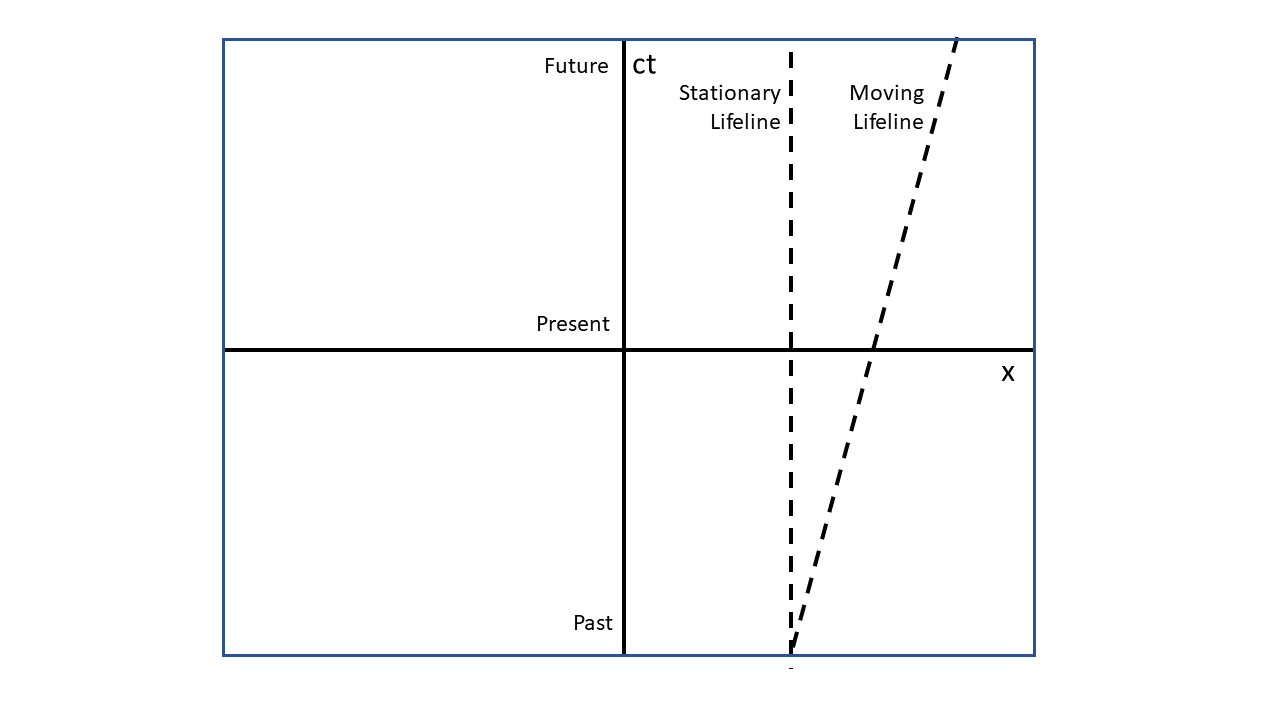Figure 2 — Minkowski diagram

These diagrams contain two axes, the x (spatial) axis and the ct (temporal) axis. The temporal axis runs from the past (bottom) to the future (top). The x axis represents one of the spatial dimensions. Concepts illustrated using only the x axis are easily extended into y and z as well. Events in spacetime are captured as points on this graph.

Two lifelines are also included in this illustration. One is for an object which is stationary. The events that make up its lifeline accumulate along the ct axis but are stationary in the x axis. The other lifeline is for an object that is moving at a constant velocity. The events making up its lifeline accumulate in both the ct and x axes. Velocity is a measure of the slope of this lifeline.

## 6.4.  Geometry and moving features

ISO 19141:2008Schema for moving features provides a framework for describing features having positions that change with time. While the standard name contains “moving features” words, the standard content is actually about moving geometries. The difference between a feature and a geometry is that the former contains arbitrary properties, and the geometry is only one of those properties. For example, a “Bridge” feature may have a geometry that describes the bridge shape, but also properties such as the maximal weight that it can support, date of last inspection, etc. The values of those properties may change with time, for example the maximal supported weight may decrease. However, ISO 19141 does not cover feature properties that change over time. Instead, dynamic or temporal properties are introduced in the OGC® Moving Features Encoding Part I: XML Core (OGC 14-083r2) standard.

Just as a line string is a sequence of points in space, a moving feature is a sequence of events in space and time. Therefore the space and time components can be handled separately — with a spatial CRS (Clause 5) defined for geometries and with dates stored separately in ISO-8601 format such as in the Moving Features CSV encoding (OGC 14-084r2). Alternatively, it is possible to combine spatiotemporal coordinates in a single four-dimensional position. This is allowed by ISO abstract models provided that a four-dimensional CRS can be associated to those positions. Underlying all geometries is the ISO 19107:2003 DirectPosition class.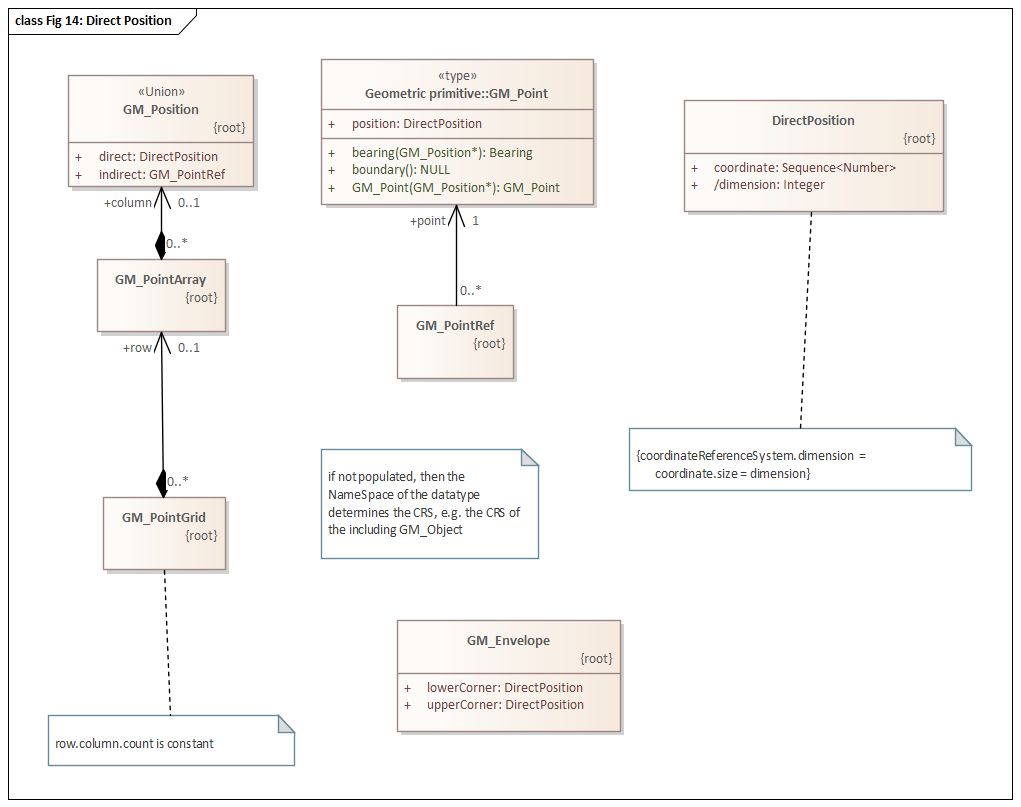Figure 3 — UML of position-related classes (source: ISO 19107)

DirectPosition has four characteristics:

• defines a coordinate tuple as a sequence of numeric values;

• the number of values in a coordinate tuple is not restricted;

• the dimension attribute specifies the number of values in the coordinate tuple; and

• The dimension value must be the same as that specified in the coordinate reference system (CRS) associated with the DirectPosition.

Therefore, a Feature geometry in four dimensions is allowed if there is a 4D Coordinate Reference System associated with the geometry. The coordinate reference system associated with a DirectPosition is defined using the CRS class from ISO 19111:2019. Temporal reference systems were initially defined in ISO 19108, but are also included in ISO 19111 since the 2019 revision. The spatial and temporal components can be combined in a single four-dimensional CRS using the CompoundCRS class. If spatial and temporal coordinate operations are also defined separately, they can be combined in a single coordinate operation using the PassThroughOperation class. Those two ISO 19111 classes are discussed in OGC 22-038 together with examples in Geographic Markup Language (GML). ISO 19107 geometries are described in more details by OGC 22-036 §5.2.4.

The two above-cited approaches are valid when space and time are independent. This is a valid approximation for non-relativist velocities. In situations where Lorentz contractions become significant (Clause 6.2), a third approach based on Minkowski space (Clause 6.3) is required. This is discussed in OGC 22-038.

### 6.4.1.  Non-linearity of time

In its review of theoretical grounds, the Testbed 18 3D+ Standards Framework Engineering Report (OGC 22-036) emphases that very few observed dynamical systems have strictly uniform time units. The transformation from one temporal CRS to another may be a complicated function even if the two CRS definitions seem compatible, for example with the same epoch and same unit of measurement. OGC 22-036 §10.3 calls for a clarification of time scales in ISO 19111. This may be a statement that the origin and calendar attributes in TemporalDatum may not be sufficient for transforming temporal coordinates without the help of an EPSG-like database. This would be consistent with geodetic coordinates, where two reference frames (datums) having different names are considered different datums even if all their attributes, such as ellipsoid, have the same values. This is because the Datum data structure cannot capture all the real-world complexity (Clause 5.1).

The temporal CRS definitions published in the OGC registry may be misleading in this aspect. For example the following definitions are “Julian date” and “truncated Julian date” respectively.

However, the above definitions are currently declared as TemporalCRS having their own TemporalDatum definitions. By definition coordinate operations between CRSs having different datums are coordinate transformations, i.e., a potentially complicated operation with stochastic errors. In reality, “Truncated Julian date” is by definition a “Julian date” with some digits truncated (more precisely, with an offset simulating a truncation). This is a coordinate conversion, an operation with theoretically no error. Coordinate conversions are by definition applied between CRSs sharing the same datum. Consequently “Truncated Julian date” should be defined as a DerivedCRS having “Julian date” as its base CRS. That way, the two temporal CRSs would share the same temporal datum, making clear that the conversion between those two CRSs is really that easy. Without this change, because the above-cited OGC registry has at least one example of datum transformation that should be exact, concluding that this simplicity may be true for many other temporal datums is tempting.

Modifying the definitions contained in the OGC CRS registry for using DerivedCRS where applicable would avoid this confusion. However, an obstacle to this change is that OGC definitions are encoded in GML, and the OGC/ISO GML Standard has not been updated to use the latest ISO 19111 revision.

### 6.4.2.  Non-rigid bodies

ISO 19141:2008 and OGC Moving Features XML and CSV encoding standards assume rigid bodies. The case of non-rigid bodies is discussed in both OGC 22-036 and OGC 22-038 and is simplified as follows

• Features are capable of movement in three dimensions. Since these Features have non-trivial three-dimensional shapes which may change over time, the movement of the Feature and the shape of the Feature are two separate properties.

• Movement is measured from the perspective of an external observer. Therefore, movement should be specified using a coordinate reference system which is external to the Feature. Example: WGS84 GeographicCRS.

• The shape of a Feature is independent of its location. The object’s geometry should be self-contained. Therefore, Feature shape should use an internal coordinate reference system. Example: EngineeringCRS with the origin at a prominent point in the Feature such as the center of mass.

For rigid bodies, coordinates of Feature geometry in the local CRS are fixed over time. They do not change regardless of any motion by the associated Feature. For non-rigid bodies, those coordinates may change over time and those changes can be described by an ISO 19111 Point Motion operation.

# 7.  Standards

The OGC 22-038 Engineering Report (ER) covers the use of ISO 19111:2019 for defining Coordinate Reference Systems attached to a spacecraft, an observatory platform, the Sun, or Earth (both ECEF and ECI cases). OGC 22-038 provides definition fragments in WKT and GML formats, together with an executable Java application reading a multi-steps chain of operations encoded in GML. The operation steps are as follows.

Voyager spacecraft ⟶ Heliocentric ⟶ ECI ⟶ ECEF ⟶ WGS84 ⟶ Tangent plane

The XML document that describes the above steps has been validated against OGC schema. The demonstration application performs a coordinate transformation using the information provided in the GML document. The parameters specified in the transformation steps are dummy, but the application is a proof of concept showing that existing OGC/ISO standards are already capable of addressing a significant part of extraterrestrial CRS needs. The ISO 19111 shortcomings, such as the absence of InertialCRS and MinkowskiCS, are summarized in OGC 22-038 and may be relatively minor additions to ISO 19111. Other possible additions are documented in sub-sections of Clause 5 in this ER.

## 7.1.  NASA SPICE

While NASA SPICE is not strictly speaking a standard, it is the software used in practice for coordinate operations between objects in space. SPICE stands for Spacecraft, Planet, Instrument, Camera-matrix, Events. The SPICE concept was defined by a group of planetary scientists as one result of the 1983 NASA-mandated Planetary Data Workshop. This section summarizes some SPICE concepts and how they relate to ISO 19111.

### 7.1.1.  Reference Frame

An ordered set of mutually orthogonal, unit length direction vectors, and possibly time dependent vectors. The frame has an associated center which must be the frame’s origin (0,0,0). When working with a body-fixed frame, this is the center of a natural body such as the sun, a planet, a satellite, a comet, or an asteroid. The body center location is specified in SPK files (SPICES proprietary software kit). SPK files also house the center location of topocentric, instruments, or spacecraft frames.

ISO 19111 equivalences: Datum and its subclasses (GeodeticReferenceFrame, TemporalDatum, etc.), except that vectors are not necessarily mutually orthogonal in the ISO standard. Furthermore, the center (anchor) is specified only by a free text in ISO 19111. However, location may be specified indirectly, relative to another frame, by a late-binding coordinate transformation.

### 7.1.2.  Inertial reference frame

A non-rotating (relative to distant stars) reference frame. The velocity may be non-zero, but the acceleration is negligible. SPICE uses the J2000 frame, which was considered the best realization until the development of ICRF (International Celestial Reference Frame). Transformation from J2000 to Earth Fixed frame uses the 1976 IAU Theory of Precession, the 1980 Nutation model, and the Greenwich Mean apparent Sidereal Time.

ISO 19111 equivalences: None. Clause 5.3 proposes adding InertialReferenceFrame and InertialCRS classes to the abstract model.

### 7.1.3.  Non-inertial reference frame

Accelerating frames, including accelerating by rotation. Candidates for utilization of this frame include the following.

• Body-fixed: associated with a natural body such as planets and satellites

• Topocentric: associated with objects near the surface or on the surface of a natural body

• Spacecraft and instrument frames: associated with objects that are attached to a spacecraft such as solar arrays, scan platforms, instruments, scanning mirrors and antennas

• Instrument: one or several frames can be associated with each instrument (applicable to a solar array and spacecraft antenna)

ISO 19111 equivalences:

• GeodeticReferenceFrame for the body-fixed case

• DerivedCRS for the topocentric case, with the geodetic reference frame as the base CRS

• EngineeringCRS for the spacecraft case

• DerivedCRS for the instrument case, with the spacecraft CRS as the base CRS

### 7.1.4.  Dynamic reference frame

A frame with a time-dependent orientation. This category does not include frames for which the orientation is provided using a spacecraft orientation or a natural body orientation.

ISO 19111 equivalences: No direct equivalence, but the same functionality can be achieved with time-varying transformation parameters. See the transformation step from ECI to ECEF in the OGC 22-038 ER. Note that ISO 19111 also has its own concept of “dynamic reference frame”, but it is used for a different problem.

### 7.1.5.  Coordinate System

A mechanism for locating points within a reference frame. SPICE uses right-handed Cartesian coordinate systems.

ISO 19111 equivalences: CoordinateSystem and its subclasses (CartesianCS, SphericalCS, etc.).

### 7.1.6.  Planetocentric coordinate system

A system where longitude is measured positive eastward, and latitude is the angle between the equatorial plane and a line from the center of the body to a given point on surface.

ISO 19111 equivalences: GeodeticCRS associated to a SphericalCS.

### 7.1.7.  Planetodetic Coordinate System

A system where longitude is the same as with planetocentric, but where latitude is the angle measured from the equatorial plane and a line perpendicular to the surface at the point of interest.

ISO 19111 equivalences: GeodeticCRS associated to an EllipsoidalCS. The GeographicCRS specialization should be used.

### 7.1.8.  State

An understanding of the reference frame and coordinate system that is going to be used. A state may be defined by position and velocity.

ISO 19111 equivalences: CoordinateMetadata. If there is a need for more metadata than what ISO 19111 provides, the CoordinateMetadata class can be extended.

## 7.2.  GeoPose

GeoPose is an OGC standard for defining reference frames anchored to the earth’s surface or within other astronomical coordinate systems. The anchor is called the “outer frame” and is defined by a CRS. The other frames are called “inner frames” and are related to the outer frame by a chain of operations. The way that inner frames are defined in GeoPose is conceptually a DerivedCRS in ISO 19111, with only a different encoding compared to WKT or GML formats. For example, consider the following GeoPose snippet:

`{  "validTime": 1674767748003,  "poseID": "https://carlsmyth.com",  "parentPoseID": "https://example.com/nodes/MarsExpress/1/Wagons/3",  "frameSpecification":  {    "authority": "https://ogc.org"",    "id": "ENGINEERINGCRS[…snip…]",    "parameters": "{\"x\": 1234567890.9876,\"y\": 2345678901.8765, \"z\": 3456789012.7654}"  },  "quaternion":  {    "x":0.0174509,"y":0.0130876,"z":-0.0002284,"w":0.9997621  }}`

Figure 4 — GeoPose snippet

NOTE  “Authority” and “ID” attributes should be used for identifying an entry in a registry such as the EPSG geodetic registry or the OGC definitions server. But in the above snippet, "authority": "https://ogc.org" means that id is a CRS standalone definition given in WKT format. It may be less confusing to replace the attribute and id pair by a different attribute such as wkt for those cases, especially since WKT is not the only CRS encoding that exists in OGC Standards.

The above snippet could be as below in ISO 19162 WKT format. For making the WKT more compact, this example uses the fact that UNIT elements in PARAMETER and ORDER elements in AXIS are optional.

`ENGINEERINGCRS["Carl Smyth"    BASEENGCRS["Mars Express Wagon 3", …snip…],    DERIVINGCONVERSION["Carl position",        METHOD["quaternion"],        PARAMETER["x",  0.0174509],        PARAMETER["y",  0.0130876],        PARAMETER["z", -0.0002284],        PARAMETER["w",  0.9997621],        PARAMETER["valid time", 1674767748003, UNIT["second", 1]]],    CS[Cartesian, 3],    AXIS["ahead (x)", forward],    AXIS["right (y)", starboard],    AXIS["down (z)", down],    ID["The voyager company", "Carl Smyth"]]`

Figure 5 — WKT equivalent for GeoPose snippet

The GeoPose Standard could be reformulated in terms of ISO 19111. For example, it would be possible to state that a GeoPose encoding is a shorthand for an ISO 19111 DerivedCRS where the coordinate system is implicitly Cartesian, and some keywords are omitted (e.g., "x":0.0174509 instead of PARAMETER["x", 0.0174509]), etc. However, the underlying abstract model could still be the ISO 19111 one.

Defining a full CRS at each step may seem unnecessarily complex. But a CRS is the combination of a reference frame (datum) and a coordinate system. In a DerivedCRS, the datum is inherited from the base CRS and does not need to be repeated. The remaining part to define is the coordinate system. That part is not necessarily a repetition, because ISO 19111 gives a choice between different kinds of coordinate systems to better match the feature geometry (Clause 5.2).

### 7.2.1.  GeoPose early-binding

A more fundamental issue with GeoPose is that it is an early-binding model. The coordinate operation that relates an inner frame to its outer frame is bundled in the frame definition itself. Consider three Coordinate Reference Systems denoted A, B, and C. Early binding models are based on the logic that if a conversion A → B exists together with a conversion A → C, then a conversion B → C can be inferred as (A → B)⁻¹ followed by (A → C), or said otherwise (B → A)(A → C). This is true when conversions are exact, but this is not accurate with transformations in the geodesic world (see ISO 19111 distinction between “conversion” and “transformation”). Another way to see the problem is to note that “if A=B and B=C then A=C” seems universal logic, but that statement is true only if the = operator represents strict equality. When that assumption is not true, then AB and BC does not necessarily imply AC. Clause 8 illustrates the problem with a use case.

Another way to describe the problem is to note that early-binding models tend to encourage the use of a “universal CRS”, which was frame A in above (B → A)(A → C) scenario. In GeoPose, the “universal CRS” is the root of a GeoPose graph. In Well-Known Text (WKT) version 1 (OGC 01-009), the “universal CRS” was WGS84 and the relationship of any CRS to that “universal” one was encoded in the TOWGS84 keyword. That keyword is deprecated and removed from ISO 19162 (a.k.a. WKT 2). Annex A gives a concrete example where TOWGS84 does not work. Another example is shown in the figure below derived from EPSG geodetic dataset version 8.9.2. There is no direct relationship between WGS84 and Japanese Geodetic Datum 2011. Note also that there are 85 different transformations between NAD27 and WGS84 (see Figure 9 for more explanation).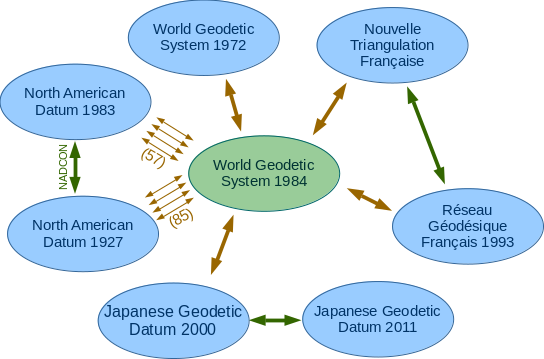Figure 6 — Example of transformations in EPSG geodetic dataset version 8.9.2

Allowing GeoPose to specify full CRS definitions at arbitrary steps does not solve the problem. The crux of the problem is that (B → A)(A → C) ≟ (B → C) is not always true. Consequently when defining frame C, it is not sufficient to provide the AC transformation. The frame definition would also need to provide the BC transformation, as well as the transformations between all other pairs of CRS involving C. The set of transformations can be reduced by excluding those that are not more accurate than the indirect path. This approach is still hardly extensible. If a frame D is added later, it should not force an update of frame C for adding the D → C transformation. It is easier to add/remove rows in a separated table of coordinate transformations, without changing the frame definitions. Especially since in software implementations, the recommendation is that frames and CRSs are immutable objects.

# 8.  Importance of datum

In this section, a use case is provided to help explain why datum information is important. From the importance of datum information, the ISO 19111 distinction between transformation and conversion is significant since the former implies a datum change while the latter is restricted to operations that do not change the datum. From the distinction between conversions and transformations, a distinction between the cases where an early-binding model can be used (i.e., when using conversions) and when a late-binding model should be used (i.e., when using transformations).

The use case is as follows. A space agency on Earth has determined that a small piece of space debris is going to collide with a station in orbit. The location of the collision is predicted in the reference frame used by Earth observatories, for example WGS84. After the collision happens, the exact location is observed by the crew onboard the space station. Then another spacecraft is sent to the station to perform repairs.

For the sake of simplicity, this ER section considers only the uncertainties caused by datum changes. All other uncertainties are ignored. This is emphasized by texts such as “assuming that Foo has infinite precision.” In the real world, the limited accuracy of Foo would be taken in account by combining its uncertainty with the datum change uncertainty. But doing so does not change the purpose of this section, which is to explain the importance of datum information.

For illustrating the uncertainty discussed in this section, consider a blue reference frame and a red reference frame. Each reference frame has its own origin, illustrated by an anchor in the following figures. In each frame, the location of 4 or 5 features are given. All coordinates in the blue frame are relative to the origin of that frame. Assuming an infinite precision, feature locations in the blue frame are represented by points.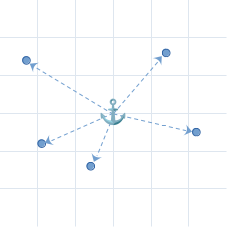Figure 7 — Locations observed from the blue frame

Likewise, all coordinates in the red frame are relative to the origin of that frame. Assuming an infinite precision, feature locations in the red frame are also represented by points.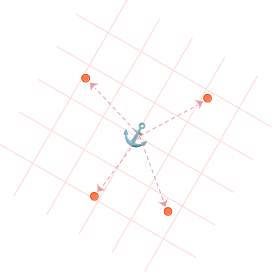Figure 8 — Locations observed from the red frame

Now if a process needs coordinates of all features in a single frame, there is a choice: either transform coordinates from the blue frame to the red frame, or conversely. Those two choices are not equivalent if the transformation between the two frames does not have infinite precision (ignoring rounding errors and other limitations of floating point arithmetic). Coordinate transformation inaccuracies are caused by stochastic errors, not by software limitations. The origin of one frame may not be well defined from the perspective of another frame. For example, because the origins of both frames are not within sight of each other. Before the satellite age, this could be because the datums were separated by a large water body or a mountain chain. This uncertainty is represented by blurred anchors in Figure 10 and Figure 11. The transformation can also be irregular, requiring interpolations in datum shift grids. This is the case of NADCON transformation for example, as shown below.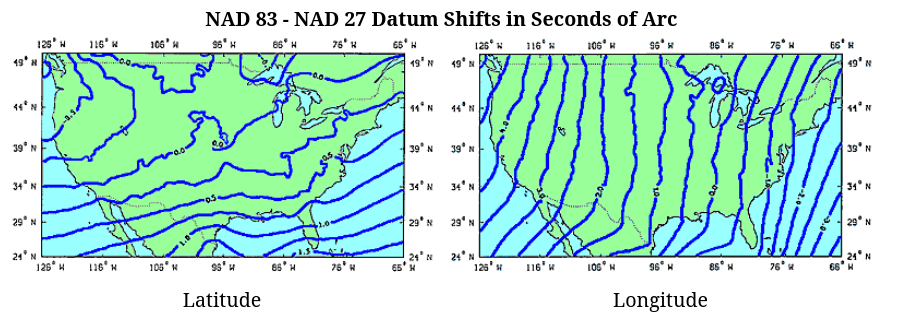Figure 9 — Source: NOAA National Geodetic Survey (NGS)

Alternatively, if a transformation is considered too complex for the desired accuracy, approximations can be used. For example, the above NADCON transformation can be replaced by a Helmert transformation (a combination of scale, rotation, and translation in geocentric coordinates). This simplification replaces the thousands of values in NADCON grids by seven parameters. Consequently, many transformations may exist between the same pair of CRSs. There are various compromises between complexity and accuracy, with different domains of validity. Indeed, the EPSG database defines approximately 85 different transformations between the same pair of CRSs (NAD27 and WGS84 in Figure 6) which are different NADCON approximations valid for different US states. These approximations can be understood as linear regressions computed using different subsets of the NADCON data illustrated in Figure 9.

If a process performs its calculation in the red reference frame, then coordinates in the blue frame must be transformed to coordinates in the red frame. But because of the transformation uncertainty, locations of blue points relative to the red frame’s origin are uncertain while the locations of red points keep their accuracy.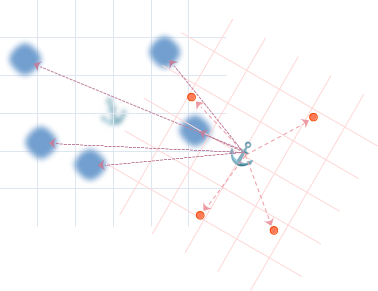Figure 10 — Locations observed from the red frame

Conversely, if the process rather performs its calculations in the blue reference frame, then the set of points that keep their accuracy and the set of points that become uncertain are the opposite of previous example.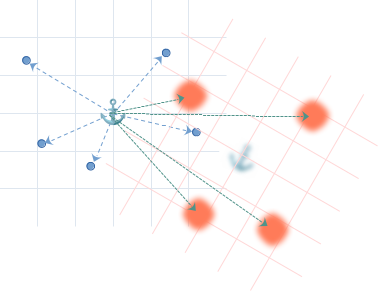Figure 11 — Locations observed from the blue frame

So even if at this stage, all points are located at the same places in both of the above figures, their uncertainties are very different depending on which datum has been chosen. This information is stored in the target Coordinate Reference System. In the next section discussion will show that not only the uncertainties are different, but the transformation results become different when more transformation steps are chained after the transformations illustrated above.

## 8.1.  Debris collision scenario

Let’s assume a small piece of space debris with a trajectory which is perfectly predicted by a numerical model working in the WGS84 reference frame. In reality the predicted trajectory would be uncertain. But that uncertainty is ignored in this section by focusing on the datum changes. This is represented by a clear line from Earth center to the space debris below, as opposed to the blur line from Earth center to the space station where a datum change will occur.

The space debris may be located using spherical coordinates (r, θ, φ) which will need to be converted to Cartesian coordinates (X, Y, Z). If the latter are geocentric coordinates relative to the same WGS84 datum, then this is a conversion, not a transformation. A conversion has no precision lost (ignoring rounding errors): the location in new Cartesian coordinate system is as accurate as the location in the previous spherical coordinate system. In ISO 19111, such conversions can be part of a DerivedCRS.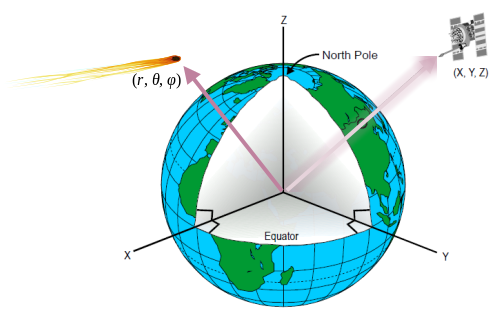Figure 12 — Debris and space station viewed from WGS84

Assume a space station is going to be hit by the space debris. The space agency wants to predict which part of the station will be hit. The hit coordinates need to be expressed in the local coordinate reference system of the station, which has its origin at the station center of mass. This implies that the (X, Y, Z) coordinates relative to WGS84 center of mass need to be transformed to (x, y, z) coordinates relative to the space station center of mass. Let’s assume that this transformation is not perfectly determined. This may be, for example, because the distance between Earth and the space station is measured by instruments affected by some kind of noise. From the WGS84 perspective, the debris location is well defined but the station location has some uncertainty as illustrated below.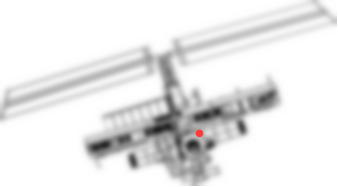Figure 13 — Predicted impact location viewed from WGS84

From the station perspective, it is the location of WGS84 center of mass which is uncertain. Consequently, the debris location, which is relative to that uncertain WGS84, is also uncertain.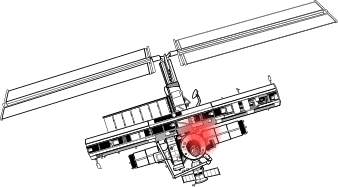Figure 14 — Predicted impact location viewed from the station engineering CRS

Now assume that the impact happened. Occupants of the space station can observe the exact hit location in the coordinates of the local CRS. Those coordinates are transmitted to another spacecraft which will come to make repairs. However, that other spacecraft needs to move its instruments according its own local CRS. Consequently, the impact coordinates need to be transformed to the spacecraft local coordinate system. There are two choices:

• transform the predicted hit location, which is relative to WGS84; or

• transform the observed hit location, which is relative to space station local CRS.

NOTE  In the introduction to this theoretical scenario, the assumption was that the debris trajectory was predicted with infinite precision in WGS84. This is of course unrealistic, but the intention is to ignore positioning errors and focus on transformation errors. In this theoretical scenario, the hit location is perfectly known in the two reference systems. The fact that this information could be used for accurately defining the transformation between the two reference systems is ignored.

Now assume that the transformation from WGS84 to the spacecraft local frame is determined by observations from the ground as illustrated by the antenna below. In this case assume that this transformation has uncertainties in the same way that the transformation from WGS84 to the space station has uncertainties. The use of WGS84 as a universal hub leads to the transformation path shown below.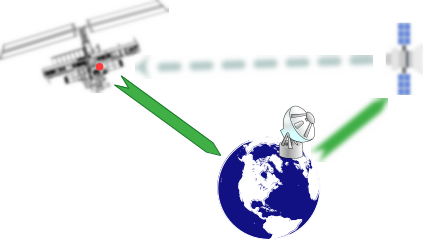Figure 15 — Hit location transformed from WGS84 frame

As stated previously, the hit coordinates in this scenario are assumed to be perfectly known in the WGS84 reference frame, so the green arrow on the left side is not blurred (to focus on the uncertainty of one transform at a time). In a more realistic scenario, which would use the hit coordinates observed by the station crew in their local reference system, the left arrow would need to be blurred as well and the uncertainties of both transforms would need to be combined.

Finally, assume that the repair spacecraft has radar and can compute its own transformation between its reference frame and the station reference frame. While not perfect (the arrow is still blurred), this transformation may be more accurate than the one passing through WGS84, for example, because both platforms are close to each other.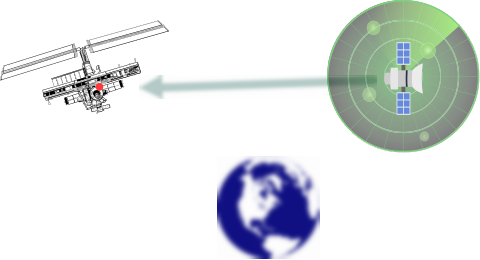Figure 16 — Hit location transformed from space station local frame

Because the transforms between the repair spacecraft and the space station were computed using different data (different radars) in the two above figures, those coordinate transformations will produce slightly different results. Consequently, even in the (unrealistic) scenario where the coordinates of an event are known with infinite precision in two different reference frames (datums), the datum of the point selected as input for the transformation will have an incidence on the numerical values of the output coordinates.

In practice, the input points will not have infinite precision. In a more realistic scenario where the predicted impact location is uncertain, there is a compromise between accepting the predicted coordinates with their uncertainty, or using the coordinates observed by the crew with the uncertainty added by the coordinate transformation (if WGS84 coordinates are desired). The best choice may seem obvious in the scenario presented in this section, but it may not be so obvious for frames far away, when data are rare or when instruments are damaged. The definition of a chain of coordinate operations should be clear about which datum are used, and where datum changes are occurring. The ISO 19111 model supports that goal.

# 9.  Key takeaways

The ubiquity of the EPSG geodetic dataset on Earth makes it easy to forget that it is at play during coordinate operations (Clause 5.1). An authority equivalent to EPSG will be needed for defining not only reference frames in space, but also the late-binding (Annex A) transformation parameters.

Some notes about possible extensions to existing OGC and ISO Standards are as follows.

• Having an attribute in CartesianCS stating whether the coordinate system is right-handed may be useful (Clause 5.2.5).

• Having an attribute in AffineCS giving the angles between pairs of axes may be useful (Clause 5.2.4).

• EllipsoidalCSs cannot be associated to EngineeringCRSs. Whether this is a problem or not has not been determined (Clause 5.2.1).

• The fact that the GML Standard has not been updated to the latest ISO 19111 revision will be an obstacle to the definition of some CRS’s.

Perhaps ISO 19111 should emphasize that two datums with different names may be related by complicated transformations even if all their attributes (ellipsoid, origin, calendar, …) have identical values. This complication applies to temporal datum as well as geodetic reference frames. The risk of confusion is illustrated by the CRS definitions published in http://www.opengis.net/def/crs/OGC/0/. This registry declares “Julian date” and “truncated Julian date” as two CRSs with two different datums, while actually the latter should be a DerivedCRS sharing the datum of the former (Clause 6.4.1). This confusion exists in the implementation of Apache SIS version 1.3 as well.

The concepts developed in GeoPose Standard and in NASA SPICE software can be mapped to ISO 19111 concepts. The ISO 19111 Standard appears to have the richest set of capabilities for expressing complex chain of coordinate operations while taking into account constraints of the physical world such as the uncertainties caused by changes of datum (Clause 8).

# Annex A(informative)Early binding versus late binding

Consider a coordinate transformation from Martinique 1938 (EPSG:4625) to RGAF09 (EPSG:5489). In an early-binding approach, the TOWGS84 parameters associated to reference frames would be as follows.

Table A.1 — Early-binding parameters

CRSTXTYTZRXRYRZΔS
Martinique 1938 (source CRS)186482151
RGAF09 (target CRS)000

The parameters for the transformation from source CRS to target CRS can be estimated by (parameters of target CRS) − (parameters of source CRS). However, this is a concatenation of two transformation steps where each step is an approximation. Chaining two approximations is not the same as performing a single approximation for the full chain. In the following table, the “early-binding” row shows the result of the above chain of two approximations, while the “late-binding” row shows the result of a single approximation as reported in EPSG database.

Table A.2 — Martinique 1938 to RGAF09 transformation parameters

MethodTXTYTZRXRYRZΔS
Early-binding186482151
Late-binding127.744547.069118.359-3.11164.9509-0.883714.1012

The consequence is an error of about 1 meter for the early-binding method, while the expected accuracy for late-binding method is about 0.1 meter. There is no way an early-binding implementation can guess with certainty the best parameters when none of the source and target CRSs are WGS84.

# Annex B(informative)Precise Positioning

## B.1.  Network Time Protocol

A standard technique for synchronizing clocks is the IETF RFC 5905 Network Time Protocol (NTP). NTP is a client-server protocol. A client requests the time from a Time Server and the server provides a response with the current time. NTP provides a precision of up to 2-64 seconds. However, precision is not accuracy. Processing the NTP packets takes time. It also takes time for the packets to traverse the network. Therefore, the accuracy of NTP can be as low as 0.01 seconds.

NTP addresses these sources of error by using a round-trip protocol. The initial request contains one timestamp – the time of the request packet transmission. The response adds two more timestamps: the time that the request was received and the time the final timestamp is the time of the response packet reception. Therefore the four times stamps are:

• t0 – request packet transmission timestamp;

• t1 – request packet reception timestamp;

• t2 – response packet transmission timestamp; and

• t3 – response packet reception timestamp

Note that the timestamps must be created as low in the protocol stack as possible to avoid additional CPU processing delays.

The difference between the client and server clocks is measured twice. First for the request packet (t₁ – t₀) and then for the response packet (t₃ – t₂). The total clock offset (θ) is the average of these two measures, mathematically defined by the formula:

$\theta =\left(\left({t}_{1}-{t}_{0}\right)+\left({t}_{2}-{t}_{3}\right)\right)/2$  (B.1)

The round-trip delay (δ) is the time spent in transit between the transmission of a request and reception of the response, mathematically defined by the formula:

$\delta =\left({t}_{3}-{t}_{0}\right)-\left({t}_{2}-{t}_{1}\right)$  (B.2)

The term t₃ – t₀ represents the total delay while the term t₂ – t₁ represents delay at the server. Since the processing time of an NTP packet should be consistent, the second term removes this delay from the measurement. The result is the delay due to network latency, the most dynamic part of the problem.

Given these concepts, the NTP protocol can be viewed as follows.

1. Issue an NTP request

3. Calculate θ

4. Calculate δ

5. IF either θ or δ is a statistical outlier, GOTO 1

6. IF δ is symmetrical (request and response delays are equal) GOTO 8

7. Adjust the local clock in the direction of Θ (incremental corrections only)

8. Wait a bit

9. GOTO 1

This approach assumes a single time server. Additional accuracy can be achieved by using multiple time servers and adjusting to the weighted average of their respective offsets.

The NTP has one source of systematic error. If the Time Servers are not accurate, then their clients will not be accurate either. This problem is addressed through an infrastructure of network time servers. At the top (stratum 0) servers are high-precision timekeeping devices such as atomic clocks. Next are the stratum 1 servers. These are servers whose system time is synchronized to within a few microseconds of their attached stratum 0 devices. Stratum 2 servers are synchronized over a network to stratum 1 servers. Stratum 3 servers are synchronized over a network to stratum 2 servers. This pattern can be replicated to stratum 15. Lower stratums naturally lead to less accuracy. Note: The time server includes its stratum level in the NTP response packet.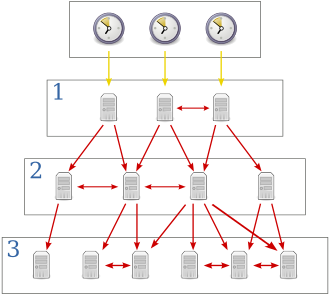Figure B.1 — Network Time Protocol servers and clients

## B.2.  Electronic Distance Measurement

Electronic Distance Measurement (EDM) devices operate by sending a laser pulse to a reflective target and measuring the time until the reflection is received back at the instrument. Distance can be calculated using the following formula:

$\rho =c\left(\Delta t/2\right)$  (B.3)

Where:

• ρ = the measured distance<

• c = the speed of light

• Δt = the interval between emission of the laser pulse and detection of its reflection

The figure below illustrates an EDM measurement. The blue sine wave indicates the emitted light. The Red sine wave indicates the reflection. The value Ρ is the distance between the EDM and the reflector.